# Math: Elementary School: 1st and 2nd Grade Quiz - Finding Halves (Questions)

This Math quiz is called 'Finding Halves' and it has been written by teachers to help you if you are studying the subject at elementary school. Playing educational quizzes is a fun way to learn if you are in the 1st or 2nd grade - aged 6 to 8.

It costs only \$12.50 per month to play this quiz and over 3,500 others that help you with your school work. You can subscribe on the page at Join Us

Halving numbers or groups of objects is often young children’s first experience of fractions. They may relate this to cutting one object in two, dividing by two or sharing one group into two equal parts. Children should recognize that for halving to be accurate, both parts should be equal in size or amount.

Do you understand halves?

1.[ ] Cut it into 4 slices [ ] Cut it into 2 equal parts [ ] Share it with some friends [ ] Eat some quickly
2.[ ] Cut some out [ ] Share them into two equal groups [ ] Put them into 4 piles [ ] Put them in a bag
3.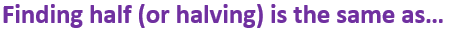[ ] Subtracting 2 [ ] Adding 2 [ ] Multiplying by 2 [ ] Dividing by 2
4.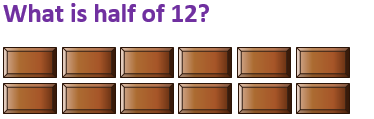[ ] 6 [ ] 12 [ ] 14 [ ] 24
5.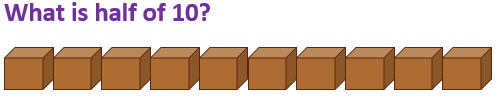[ ] 8 [ ] 5 [ ] 20 [ ] 35
6.[ ] 16 [ ] 14 [ ] 22 [ ] 10
7.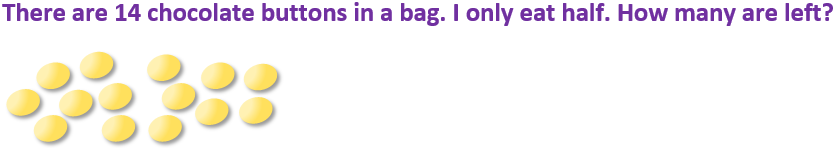[ ] 7 [ ] 6 [ ] 5 [ ] 10
8.[ ] 14 [ ] 10 [ ] 16 [ ] 9
9.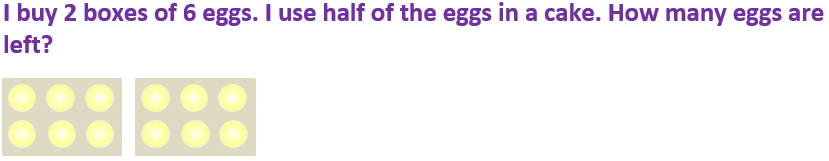[ ] 2 [ ] 16 [ ] 6 [ ] 4
10.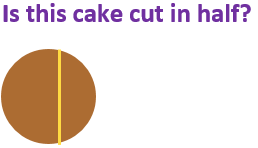[ ] Yes, there is a line down the middle [ ] Yes, it has been shared between two people [ ] Yes, there are two pieces [ ] No, the two pieces aren’t the same sizeMath: Elementary School: 1st and 2nd Grade Quiz - Finding Halves (Answers)
1.[ ] Cut it into 4 slices [x] Cut it into 2 equal parts [ ] Share it with some friends [ ] Eat some quickly
The two halves should be exactly the same size
2.[ ] Cut some out [x] Share them into two equal groups [ ] Put them into 4 piles [ ] Put them in a bag
Each group should have exactly the same number of stars
3.[ ] Subtracting 2 [ ] Adding 2 [ ] Multiplying by 2 [x] Dividing by 2
Halving means sharing into 2 equal groups or parts
4.[x] 6 [ ] 12 [ ] 14 [ ] 24
12 ÷ 2 = 6, so half of 12 is 6
5.[ ] 8 [x] 5 [ ] 20 [ ] 35
10 shared into 2 equal groups gives 5 in each group, so half of 10 is 5
6.[ ] 16 [ ] 14 [ ] 22 [x] 10
Sharing 20 into two equal groups would give 10 in each group
7.[x] 7 [ ] 6 [ ] 5 [ ] 10
Half of 14 is 7
8.[ ] 14 [ ] 10 [ ] 16 [x] 9
Half of 10 is 5, half of 8 is 4. 5 + 4 = 9
9.[ ] 2 [ ] 16 [x] 6 [ ] 4
Using half of the eggs would be the same as using one box only
10.[ ] Yes, there is a line down the middle [ ] Yes, it has been shared between two people [ ] Yes, there are two pieces [x] No, the two pieces aren’t the same size
Halves of a shape must be identical